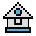Home

# FOR ... NEXT Statements

#### Purpose:

To execute a series of instructions a specified number of times in a loop.

#### Syntax:

```FOR variable=x TO y [STEP z]
.
.
.
NEXT [variable][,variable...]```

variable is used as a counter.

x,y, and z are numeric expressions.

STEP z specifies the counter increment for each loop.

The first numeric expression (x) is the initial value of the counter. The second numeric expression (y) is the final value of the counter.

Program lines following the FOR statement are executed until the NEXT statement is encountered. Then, the counter is incremented by the amount specified by STEP.

If STEP is not specified, the increment is assumed to be 1.

A check is performed to see if the value of the counter is now greater than the final value (y). If it is not greater, GW-BASIC branches back to the statement after the FOR statement, and the process is repeated. If it is greater, execution continues with the statement following the NEXT statement. This is a FOR-NEXT loop.

The body of the loop is skipped if the initial value of the loop times the sign of the step exceeds the final value times the sign of the step.

If STEP is negative, the final value of the counter is set to be less than the initial value. The counter is decremented each time through the loop, and the loop is executed until the counter is less than the final value.

#### Nested Loops:

FOR-NEXT loops may be nested; that is, a FOR-NEXT loop may be placed within the context of another FOR-NEXT loop. When loops are nested, each loop must have a unique variable name as its counter.

The NEXT statement for the inside loop must appear before that for the outside loop.

If nested loops have the same end point, a single NEXT statement may be used for all of them.

The variable(s) in the NEXT statement may be omitted, in which case the NEXT statement will match the most recent FOR statement.

If a NEXT statement is encountered before its corresponding FOR statement, a "NEXT without FOR" error message is issued and execution is terminated.

#### Examples:

The following example prints integer values of the variable I% from 1 to 10 in steps of z. For fastest execution, I is declared as an integer by the % sign.

```10 K=10
20 FOR I%=1 TO K STEP 2
30 ĀĀPRINT I%
40 NEXT
RUN
Ā1
Ā3
Ā5
Ā7
Ā9```

In the following example, the loop does not execute because the initial value of the loop exceeds the final value. Nothing is printed by this example.

```10 R=0
20 FOR S=1 TO R
30 ĀĀPRINT S
40 NEXT S```

In the next example, the loop executes 10 times. The final value for the loop variable is always set before the initial value is set.

```10 S=5
20 FOR S=1 TO S+5
30 ĀĀPRINT S;
40 NEXT
RUN
Ā1 2 3 4 5 6 7 8 9 10```